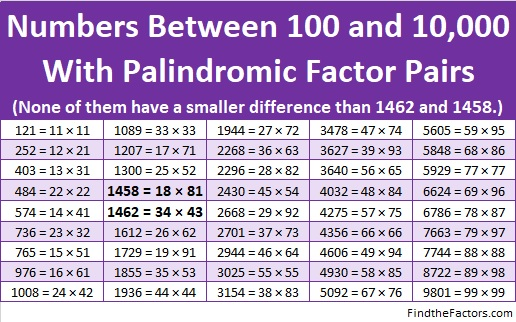# 1462 Has a Palindromic Factor Pair

There are only forty-five numbers between 100 and 10,000 that have palindromic factor pairs. That’s really not very many.

1462 is one of those numbers. That is pretty amazing, all by itself, but 1462 is also only four numbers more than another number on the list. That’s even more amazing!Here are some more facts about the number 1462:

• 1462 is a composite number.
• Prime factorization: 1462 = 2 × 17 × 43
• 1462 has no exponents greater than 1 in its prime factorization, so √1462 cannot be simplified.
• The exponents in the prime factorization are 1, 1, and 1. Adding one to each exponent and multiplying we get (1 + 1)(1 + 1)(1 + 1) = 2 × 2 × 2 = 8. Therefore 1462 has exactly 8 factors.
• The factors of 1462 are outlined with their factor pair partners in the graphic below.1462 is the hypotenuse of one Pythagorean triple:
688-1290-1462 which is (8-15-17) times 86